## Introduction

This example demonstrates how the results of a QUAKE/W analysis can be used in conjunction with SLOPE/W to estimate the permanent deformations that may occur as a result of the inertial forces associated with an earthquake.

This type of permanent deformation analysis is referred to as a Newmark analysis. The premise of a Newmark analysis is that during an earthquake, there will be short moments in time when the inertial forces (mass times acceleration) plus the initial static forces will exceed the available shear resistance and during these times the temporary loss of stability will lead to un-recoverable deformations. The accumulation of the un-recoverable deformations will manifest itself as permanent deformation after the shaking has stopped.

The details of the implementation for this type of analysis are presented in the SLOPE/W Engineering Book. Only the highlights of an illustrative example are presented here.

## Numerical Simulation

A similar example is presented as part of the SLOPE/W group of examples. A variation of the SLOPE/W example is presented to enhance the QUAKE/W example list and for convenient reference when one is examining the QUAKE/W features and capabilities. Figure 1 presents the simple slope used in this illustrative analysis.##### Figure 1. Problem configuration.

The first step is to establish the in situ state of stress in the ground that exists before the earthquake occurs and shakes the ground. In this case, this is done with the QUAKE/W Initial static type of analysis, which basically involves doing a simple gravity turn-on type of analysis.

The next step is to subject the slope to an earthquake with a time history record shown in Figure 2. The peak acceleration is 0.4 g at about the 2-sec mark in the 10 seconds of shaking. The earthquake data for this record has a constant time interval of 0.02 seconds. For 10 seconds, this means there are 500 data points. The output data is saved to file only every 10th time step plus at 20 of the highest peaks in the record.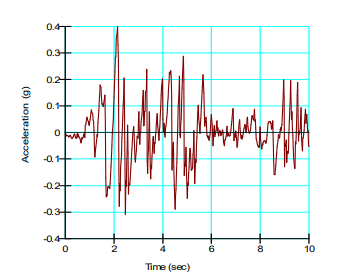##### Figure 2. Earthquake time history record.

Only linear-elastic soil properties are used here in this simple illustrative example. The total unit weight is defined as 18 kN/m3 with a Poisson’s ratio of 0.334 and a Damping Ratio of 0.1. The Gmax is defined at a constant 20,000 kPa. The left and right boundary conditions are fixed in the x-direction in the Initial Static analysis, but then fixed only in the y-direction in the Shaking analysis.

Slope stability analyses have been developed for both the initial static analysis to determine the initial factor of safety and again during the shaking analysis to determine the factor of safety versus time during the earthquake.

## Results and Discussion

The results of the Initial Static analysis are the most conveniently inspected by creating contour plots of the various stress components. Figure 3 shows a typical example.##### Figure 3. Maximum static shear stress contours.

Under static conditions, the factor of safety is about 1.13 (Figure 4).##### Figure 4. Stability of slope under static conditions.

Within the red band in Figure 4, the Factor of Safety varies between 1.129 and 1.229 – about a 10% variation.

It is intuitively obvious that the earthquake inertial forces will pull the slope in both the up-slope direction and in the down-slope direction. This is illustrated in Figure 5 and in Figure 6. Figure 7 shows the lateral back and forth displacement of the slope crest.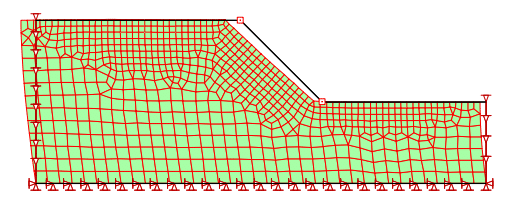##### Figure 5. Displacement at the 1.60 second mark (100x exaggeration).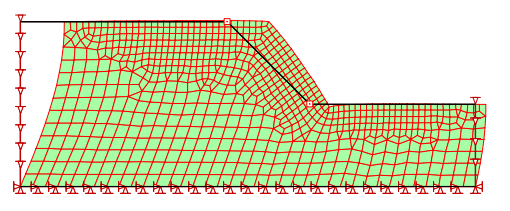##### Figure 6. Displacement at the 5.40 second mark (100x exaggeration).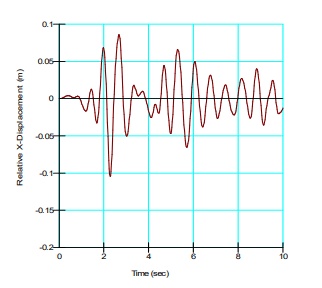##### Figure 7. Lateral back and forth movement of the slope crest during the shaking.

From the illustrations in the above figures, it is easy to visualize that the factor of safety will go up and down during the shaking. The objective is to determine how many times and for how long the factor of safety is below 1.0 when there is a momentary loss of stability.

The details of how finite element-computed stresses can be used in SLOPE/W to compute safety factors are presented in the SLOPE/W Engineering Book. It is sufficient to say here that for each trial slip surface, SLOPE/W computes the mobilized shear and normal stress at the base of each slice. The normal stress is then used in conjunction with the specified strength parameters to compute the available shear resistance at the base of each slice. A ratio of the summation of the available shear resistance to the summation of the mobilized shear is taken as the safety factor.

For a Newmark-type analysis, the soil strength is deemed to remain constant during the shaking (undrained behavior). The strength is therefore computed on the basis of the initial static stresses. Only the mobilized shear is re-computed for each time step that the data is saved to file.

The permanent deformation is computed for 18 trial slip surfaces in this example.

The largest computed deformations occur for Slip Surface 2. The static factor of safety for this slip surface is 1.197, as shown in Figure 8. Note, it is not the slip with the lowest static factor of safety.##### Figure 8. Slip surface with the largest deformation.

Figure 9 presents the variation in the safety factors during the shaking for this slip surface. Note that there are moments when the factor of safety is less than unity (1.0), indicating that there are moments when the slope is temporarily unstable.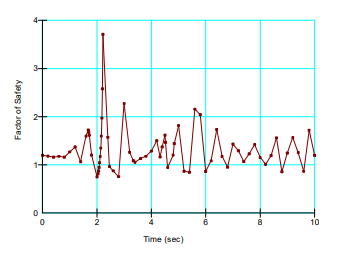##### Figure 9. Variation in safety factors during the shaking.

SLOPE/W computes the so-called yield acceleration from the data in Figure 10. The yield acceleration occurs where the factor of safety is 1.0. In this case, the yield acceleration is about 0.10g.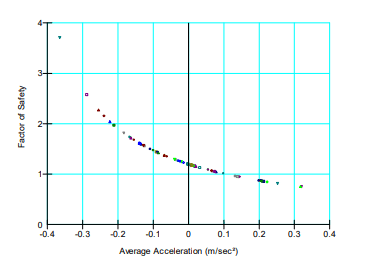##### Figure 10. Factor of safety versus the average acceleration.

Once the yield acceleration is known, SLOPE/W integrates the area under the curve in Figure 11, where the acceleration is greater than the yield acceleration, which in this case is 0.10 g. The integrated area gives the velocity as shown in Figure 12.##### Figure 11. Average acceleration with time.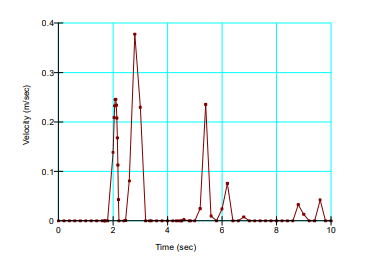##### Figure 12. Velocity during moments of temporary loss of stability.

Integrating the area under the velocity curve gives the accumulated deformation, as shown in Figure 13.##### Figure 13. Accumulated deformations during moments of temporary loss of stability.

In summary, this analysis indicates that there could be approximately 0.28 m permanent deformation of this slope if it were to be subjected to the earthquake.

The movement would be along the trial slip surface. In this case, with the slip surface being so steep, most of the movement would manifest itself as vertical movement, although there could be a slight amount of rotational movement as well.

## Summary and Conclusions

It is vitally important to be mindful of the fact that a Newmark analysis involves only inertial forces and is applicable only to cases where there will not be a significant loss in shear strength and the generation of significant excess pore-water pressures.

The Newmark analysis is very computationally demanding. For each trial slip surface, SLOPE/W computes a factor of safety for each QUAKE/W time step for which the data was saved. The computing time multiplies very quickly if there are a lot of QUAKE/W data files and many trial slip surfaces.

The recommended procedure is to establish the critical slip surface first, based on the initial static stresses. Then select a few trial slip surfaces around the static critical slip for a Newmark deformation analysis.

The QUAKE/W – SLOPE/W integration makes it possible to do a Newmark-type permanent deformation analysis for any potential slip surface.